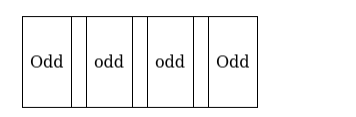# How many numbers can be formed with the digits 2, 3, 4, 5, 4, 3, 2

Question:

How many numbers can be formed with the digits 2, 3, 4, 5, 4, 3, 2 so that the odd digits occupy the odd places?

Solution:

The table shows the places where the odd digits can be placedThere are 4 places

And 3 odd digits out of which 2 are of the same kind

Choose any 3 places out of the four places in ${ }^{4} \mathrm{C}_{3}$ ways $=4$ ways

In each way, the 3 digits can be placed in $\frac{3 !}{2 !}$ ways $=3$ ways

$\Rightarrow$ Total number of ways in which odd digits occupy odd places $=4 \times 3=12$

Now there are 4 remaining digits out of which 2 are same of 1 kind, and 2 are same as another kind

$\Rightarrow$ They can be arranged in the remaining places in $\frac{4 !}{2 ! 2 !}=6$ ways

$\Rightarrow$ Total number of numbers where odd digit occupies odd places $=12 \times 6=72$

There are 72 such numbers